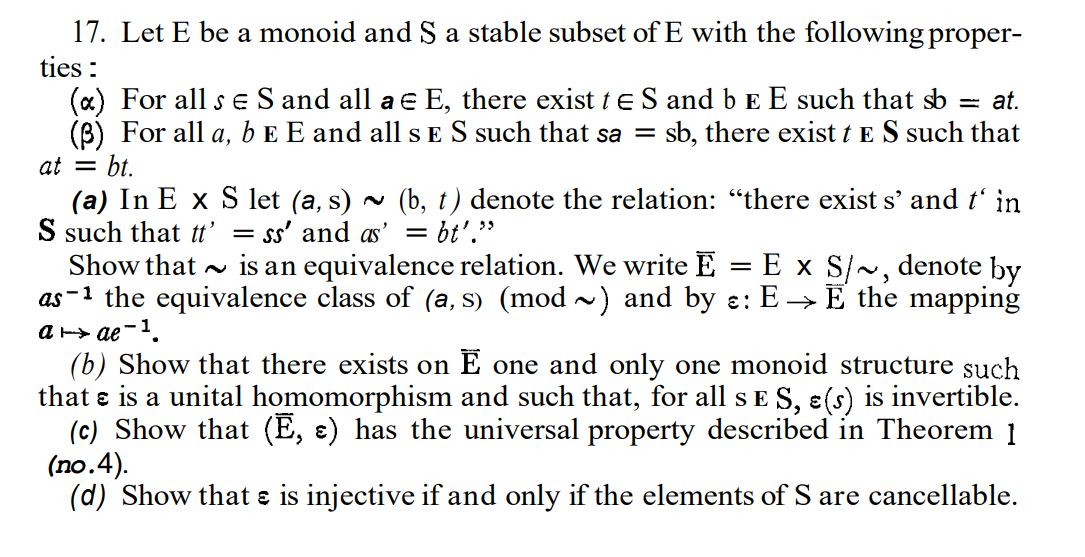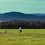# "Right denominator set" | How to show that the relation is transitive?

This problem comes from N. Bourbaki - Algebra I - Chapter 1.Let $E$ be a monoid and $S\subset E$ a submonoid satisfying the following properties:

$(1)$ For every $a\in E$ and $s\in S$, there exists $t\in S$ and $b\in E$ such that $sb=at$.

$(2)$ For every $a,b\in E$ and $s\in S$ such that $sa=sb$, there exists $t\in S$ such that $at=bt$.

Define a relation $(a,s)\sim (b,t)$ on $E\times S$ to mean that there exists $s',t'\in S$ such that $tt'=ss'$ and $as'=bt'$.

Show that the above $\sim$ is an equivalence relation on $E\times S$.

My first attempt (to show the transitivity) :

Let $(a,s)\sim (b,t)$ and $(b,t)\sim (c,u)$. Then

$\exists s',t'\in S\text{ s.t. } tt'=ss',as'=bt'$

$\exists t'',u'\in S\text{ s.t. } uu'=tt'',bt''=cu'$

By $(1)$, for $(t',t'')\in E\times S$, there exists $(v,w)\in E\times S$ s.t. $t'w=t''v$. Thus

$as'w=bt'w=bt''v=cu'v$

$ss'w=tt'w=tt''v=uu'v$

Since $s',w\in S$ and $S$ is a monoid, we have $s'w\in S$. But $u'v$ may not belong to $S$. Besides, the second condition has not been used. So I got stuck here. And my other attempts also failed because some element may not belong to $S$.

Update. My second attempt (a similar method in T.Y.Lam's book Modules and Rings - Theorem 10.6) :

Let $(a,s)\sim (b,t)$ and $(b,t)\sim (c,u)$. Then $\exists s',t'\in S\text{ s.t. } tt'=ss',as'=bt'$ $\exists t'',u'\in S\text{ s.t. } uu'=tt'',bt''=cu'$ First we try to obtain a common denominator''. Note that $tt'\in S,tt''\in S$, by condition $(1)$ we have $tt''v=tt'w\in S$ for some $v\in E$ and $w\in S$.

Now by condition $(2)$ we have $t''v\widehat{t}=t'w\widehat{t}\in S$ for some $\widehat{t}\in S$.

Thus we get $c(u'v\widehat{t})=(cu')v\widehat{t}=(bt'')v\widehat{t} =b(t''v\widehat{t}) =b(t'w\widehat{t})=(bt')w\widehat{t}=(as')w\widehat{t}=a(s'w\widehat{t})$ Besides, we have $u(u'v\widehat{t})=(uu')v\widehat{t}=(tt'')v\widehat{t}=t(t''v\widehat{t}) =t(t'w\widehat{t})=(tt')w\widehat{t}=(ss')w\widehat{t}=s(s'w\widehat{t})$ Since $s',w,\widehat{t}\in S$ and $S$ is a submonoid of $E$, $s'w\widehat{t}\in S$. The remaining obstacle is that I cannot decide whether $u'v\widehat{t}\in S$ holds or not.

Any comments or suggestions are welcome.Note by Haosen Chen
8 months, 3 weeks ago

This discussion board is a place to discuss our Daily Challenges and the math and science related to those challenges. Explanations are more than just a solution — they should explain the steps and thinking strategies that you used to obtain the solution. Comments should further the discussion of math and science.

When posting on Brilliant:

• Use the emojis to react to an explanation, whether you're congratulating a job well done , or just really confused .
• Ask specific questions about the challenge or the steps in somebody's explanation. Well-posed questions can add a lot to the discussion, but posting "I don't understand!" doesn't help anyone.
• Try to contribute something new to the discussion, whether it is an extension, generalization or other idea related to the challenge.
• Stay on topic — we're all here to learn more about math and science, not to hear about your favorite get-rich-quick scheme or current world events.

MarkdownAppears as
*italics* or _italics_ italics
**bold** or __bold__ bold
- bulleted- list
• bulleted
• list
1. numbered2. list
1. numbered
2. list
Note: you must add a full line of space before and after lists for them to show up correctly
paragraph 1paragraph 2

paragraph 1

paragraph 2

[example link](https://brilliant.org)example link
> This is a quote
This is a quote
    # I indented these lines
# 4 spaces, and now they show
# up as a code block.

print "hello world"
# I indented these lines
# 4 spaces, and now they show
# up as a code block.

print "hello world"
MathAppears as
Remember to wrap math in $$ ... $$ or $ ... $ to ensure proper formatting.
2 \times 3 $2 \times 3$
2^{34} $2^{34}$
a_{i-1} $a_{i-1}$
\frac{2}{3} $\frac{2}{3}$
\sqrt{2} $\sqrt{2}$
\sum_{i=1}^3 $\sum_{i=1}^3$
\sin \theta $\sin \theta$
\boxed{123} $\boxed{123}$

## Comments

Sort by:

Top Newest

I think you started in the right way. The only extra leverage I can see is that, since $t'$ and $t''$ are both in $S,$ you can find solutions to your equation $t'w = t''v$ in two ways: one with $v_1 \in E$ and $w_1 \in S,$ and another with $v_2 \in S$ and $w_2 \in E.$

I played around with this a little--I even managed to get to a point where I used (2) in a natural-looking way--but I couldn't get an expression involving only things in $S.$

- 8 months, 2 weeks ago

Log in to reply

×

Problem Loading...

Note Loading...

Set Loading...